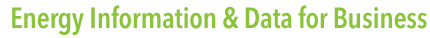Energy Information, data and forecasts for business##FREE ENERGY DATA# The cost of electricity in the future, the price of renewable energy

The cost of electricity is a complex calculation. Using a number of assumptions it is possible to calculate the cost of producing electricity in a fixed situation but there are still external variables, especially in a lifetime cost calculation, which an owner has to evaluate with additional risk and sensitivity analysis. These include political factors such as the impact of the two oil crises on the 1970s which have had a permanent effect on many countries’ energy policies, climate considerations, financial trends and many others. There are many occasions when the cheapest or most convenient solution is not the best. However, we have to have some cost estimation as a basis for the decision. Two approaches are most commonly used, overnight cost and levelised cost.
Overnight cost is the cost of a construction project if no interest was incurred during construction, as if the project was completed “overnight.” An alternate definition is: the present value cost that would have to be paid as a lump sum up front to pay for a construction project completely. When describing power plants, the unit of measure typically used when citing the overnight cost of a power plant is \$/kW. For example, the overnight cost of a nuclear plant might be \$1,200/kW, so a 1,000MW plant would have an overnight cost \$1.2 billion. Interest on the \$1.2 billion spent during construction would be extra.
Levelised cost (LCOE –levelised cost of electricity) is the cost of generating electricity using the simple levelised average (unit) lifetime cost method using discounted cash flow (DCF). This takes into account many factors; construction cost, interest rate, fuel cost, carbon cost, the rate of replacement for plant, decommissioning cost, fixed O&M costs, load factors and other cost components. It is quite simply the total cost, discounted to present day values of every element of forseeable cost in a plant’s lifetime. It is a useful method of comparing the cost of generating electricity with different technologies.